Home    |    Teacher    |    Parents    |    Glossary    |    About UsEmail this page to a friendResources· Cool Tools · Formulas & Tables · References · Test Preparation · Study Tips · Wonders of MathSearchPerimeter Formulas (Math | Geometry | Perimeter Formulas)

(pi =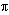= 3.141592...)

Perimeter Formulas
The perimeter of any polygon is the sum of the lengths of all the sides.

### Be careful!! Units count. Use the same units for all measurements. Examples

square = 4a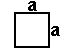rectangle = 2a + 2b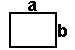triangle = a + b + c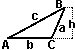circle = 2pi r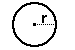circle = pi d (where d is the diameter)

The perimeter of a circle is more commonly known as the circumference.Units

Be sure to only add similar units. For example, you cannot add inches to feet.

For example, if you need to find the perimeter of a rectangle with sides of 9 inches and 1 foot, you must first change to the same units.

perimeter = 2 ( a + b)

INCORRECT
perimeter = 2(9 + 1) = 2*10 = 20

CORRECT
perimeter = 2( 9 inches + 1 foot)
= 2( 3/4 foot + 1 foot )
= 2(1 3/4 feet)
= 3 1/2 feet